Definition

Chapter 2 Class 9 Polynomials
Concept wise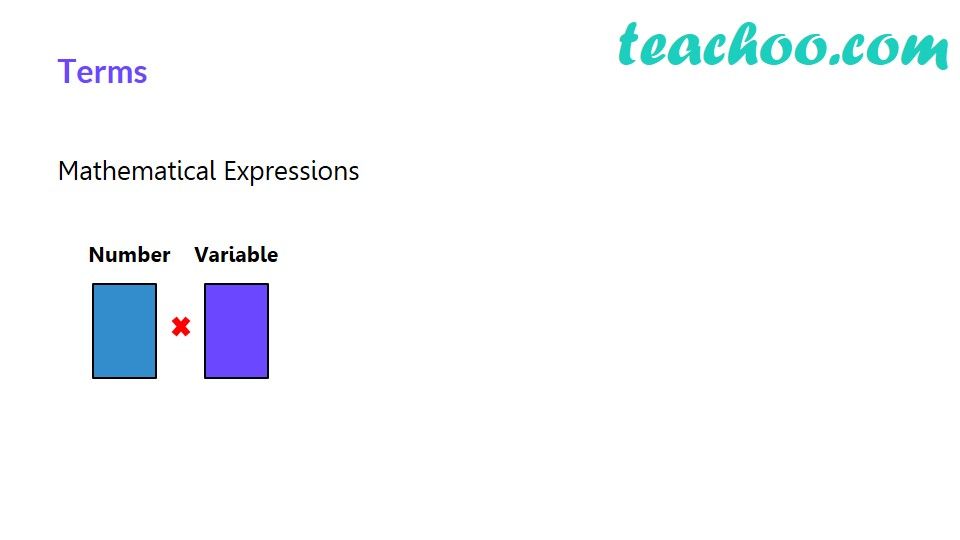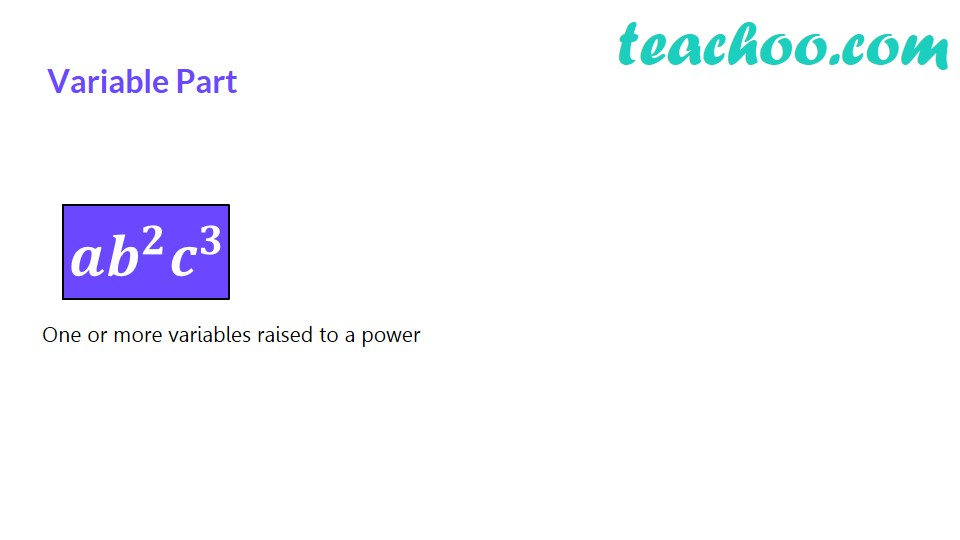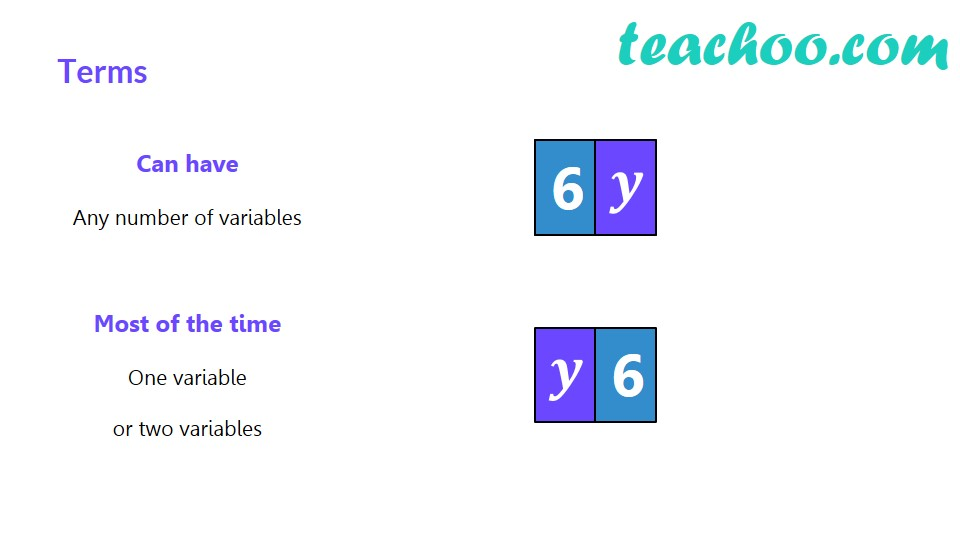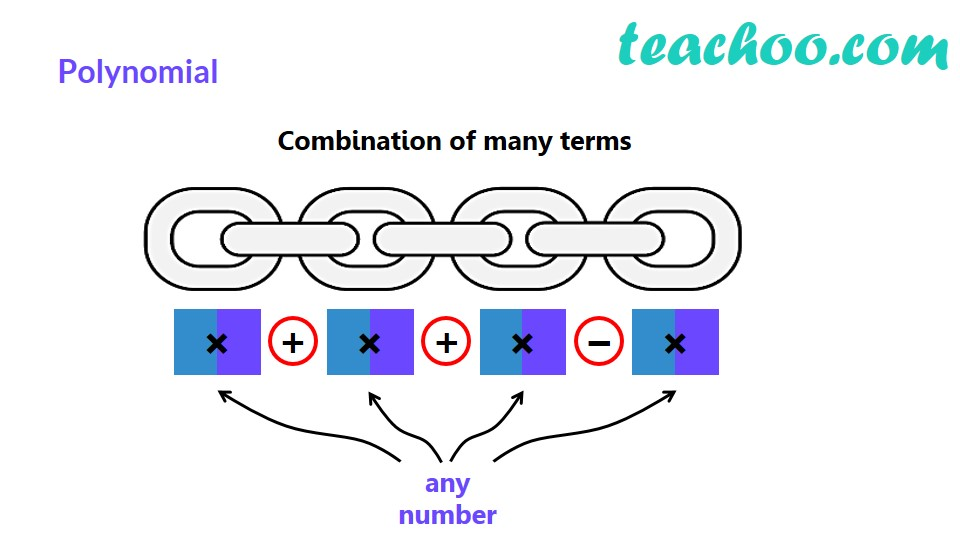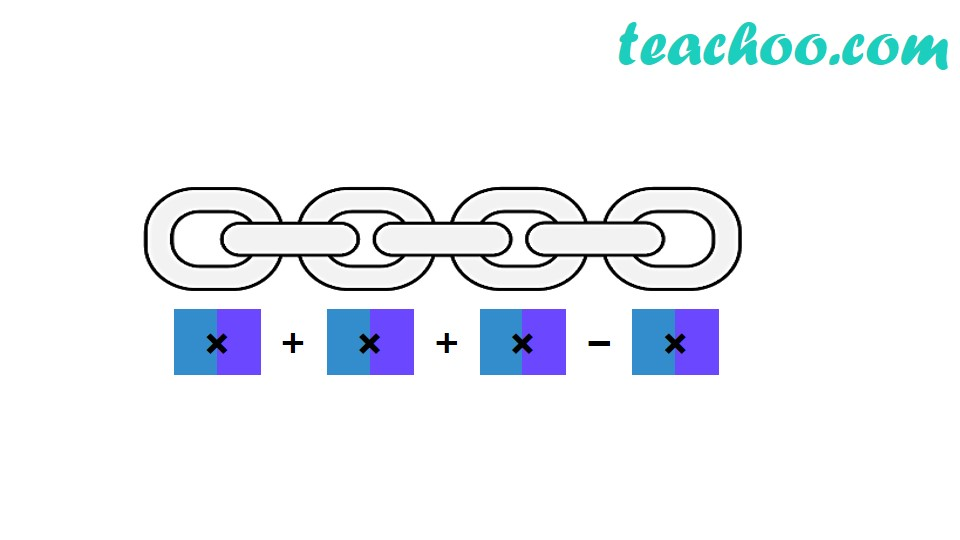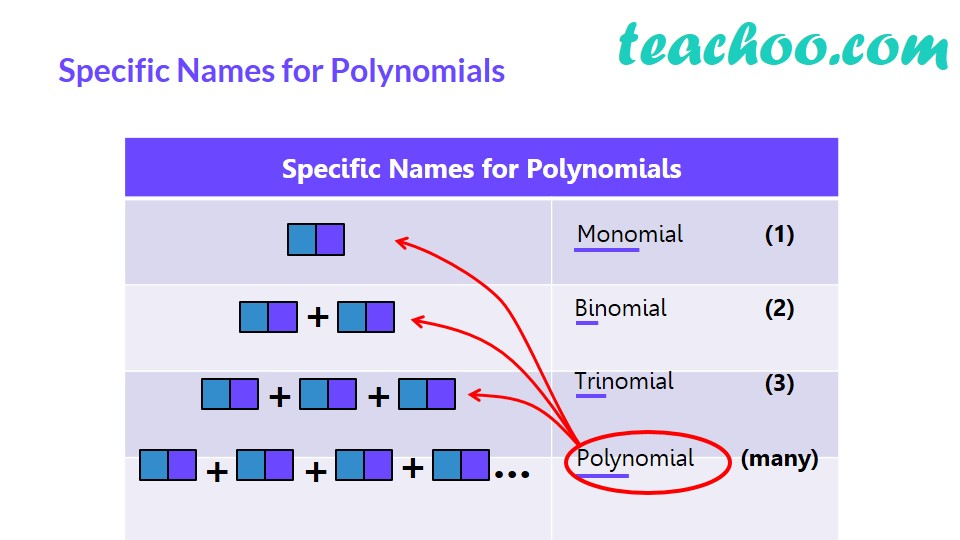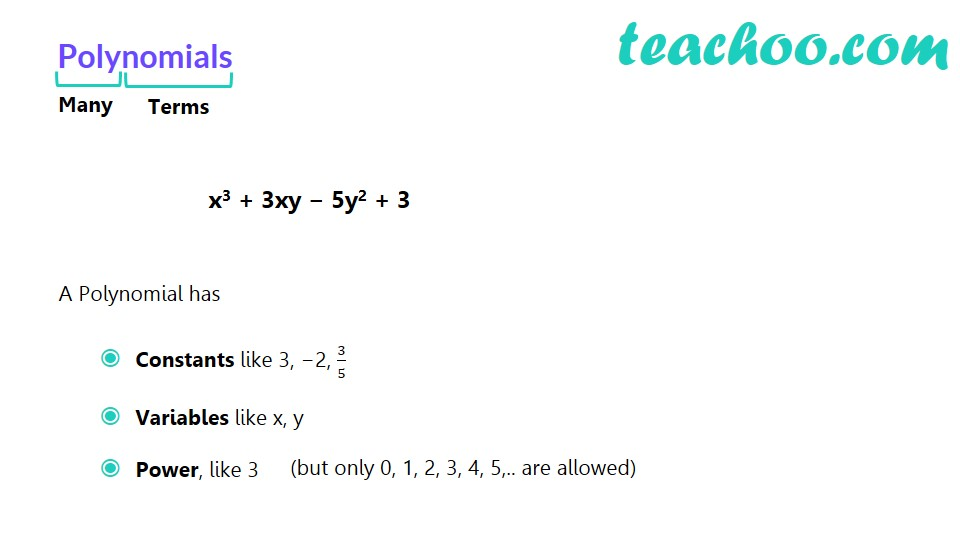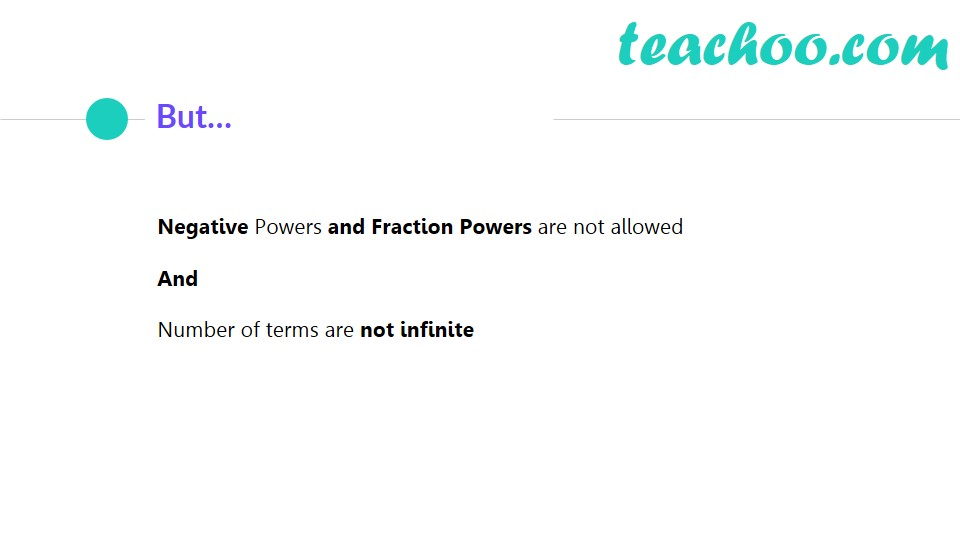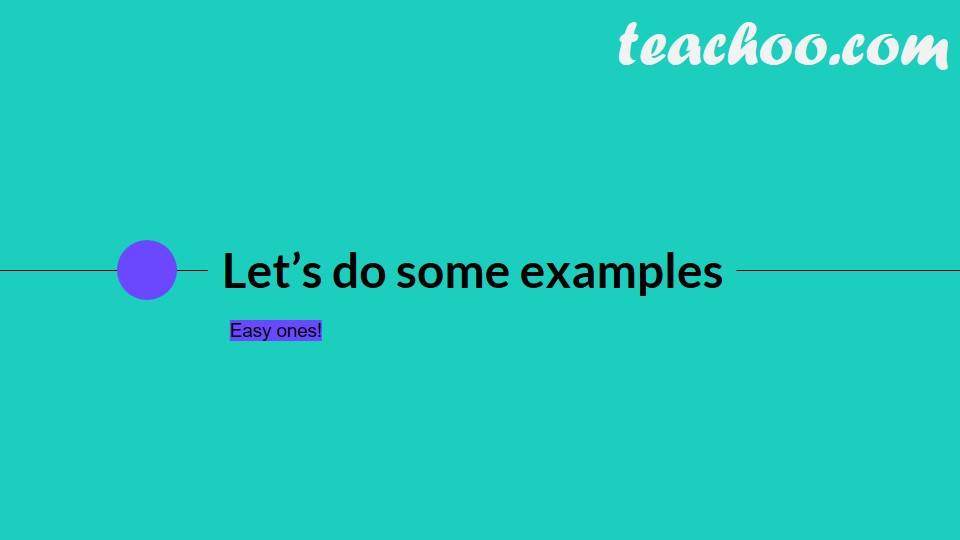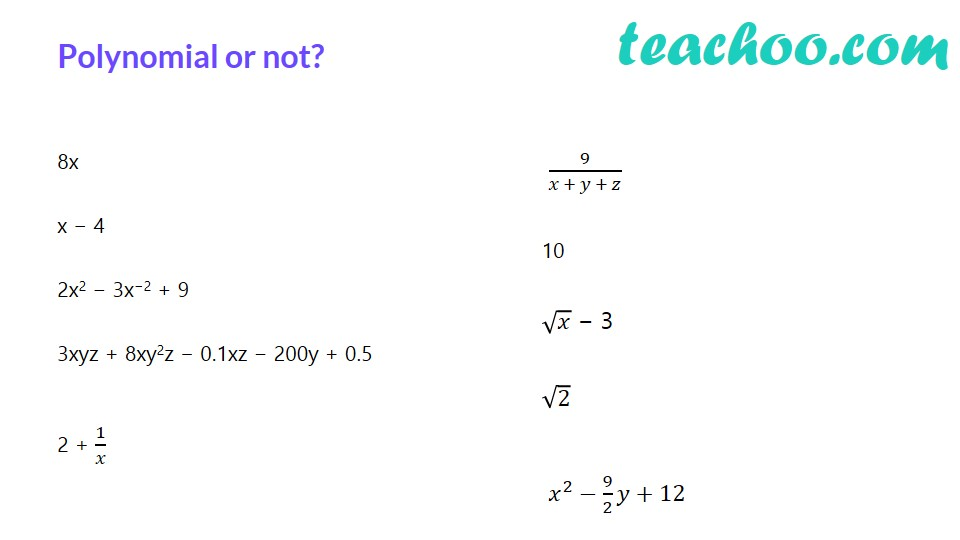Equations of the type

x 3 − 3x 2 + 4x + 10

are called polynomials.

Here,

x 3 , −3x 2 , 4x , 10 are called terms of polynomials.

A Polynomial has

• Variables like x, y with powers as whole numbers (0, 1, 2, 3, ....). If Power is not a whole number , the equation is not a polynomial.
• constants like 10

## Polynomial in one variable

In this chapter,

We will be studying polynomials which are in one variable, i.e. only has x or y, but not both.

Example - x 2 + 2x - 3 is a polynomial in one variable as there is only x

But x 2 + 2y - 3x = 0 is not a polynomial in one variable as there is both x and y.

Is x 2 + 24y + 1 a  polynomial in one variable?

-a-

Since there are two variables – x and y

It is not a polynomial in 1 variable

-ea-

Is y 4 – 10 a polynomial in one variable?

-a-

y 4 – 10 = y 4 – 10y 0

Here power are 4 and 0.

Since all powers are whole numbers, it is a polynomial

And since there is only one variable y,

It is a polynomial in one variable

-ea-

Is 5x -2 + 1 a polynomial in one variable?

-a-

Since power is -2

And -2 is not a whole number,

It is not a polynomial in one variable

-ea-

Is  3x 1/2 + 2 a polynomial in one variable?

-a-

Since power is 1/2

And 1/2 is not a whole number,

It is not a polynomial in one variable

-ea-

Is 3 a polynomial in one variable?

-a-

3 = 3x 0

Since power is 0

And 0 is a whole number,

It is a polynomial.

And since there is only 1 variable x,

It is a polynomial in one variable

-ea-

Note:

Constant numbers are also polynomials.

Example: 2, 3, 100, -988999 are all polynomials

Introducing your new favourite teacher - Teachoo Black, at only ₹83 per month